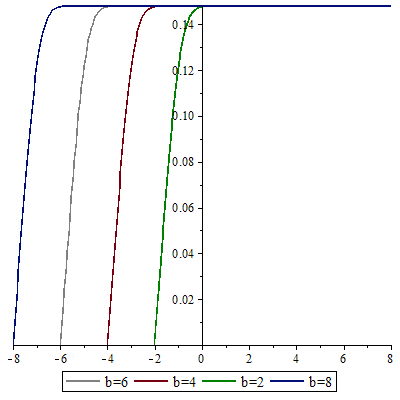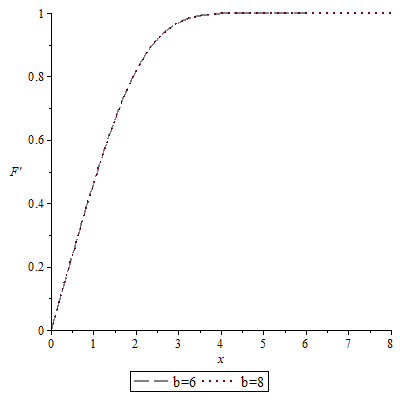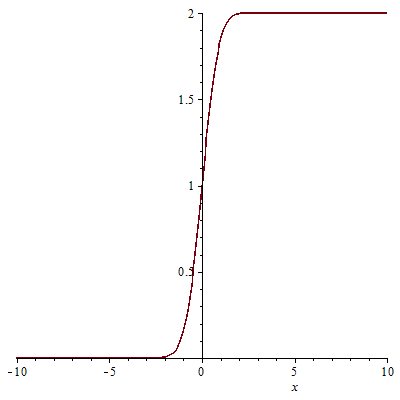## 195 Reputation

12 years, 134 days

Amir

## @Preben Alsholm  yes. it works ver...

yes. it works very well.

## @Preben Alsholm  yes. it works ver...

yes. it works very well.

## @Preben Alsholm  Dear Preben Alsho...

Dear Preben Alsholm

but fsolve is a little inefficient (get long tims) i think. i try it with some parameters that i know the answer.

with this command

fsolve({pC(c1,t1),pT(c1,t1)},{c1=-5.55..-5.3,t1=-.67..-0.66});

but it takes long times (30s). as you can see the domain [-5.55..-5.3] and [-.67..-0.66] is little and 30s is very long for this. for the some parameters that i dont know the solution i must increase the domain and it takes a long time. i want to know is there another idea, that gets less time, or parallel fsolve or ...

## @Preben Alsholm  Dear Preben Alsho...

Dear Preben Alsholm

but fsolve is a little inefficient (get long tims) i think. i try it with some parameters that i know the answer.

with this command

fsolve({pC(c1,t1),pT(c1,t1)},{c1=-5.55..-5.3,t1=-.67..-0.66});

but it takes long times (30s). as you can see the domain [-5.55..-5.3] and [-.67..-0.66] is little and 30s is very long for this. for the some parameters that i dont know the solution i must increase the domain and it takes a long time. i want to know is there another idea, that gets less time, or parallel fsolve or ...

hi

in the hedayati's problem -6 stands for -b. the actual differential equations is

diff(F(x), x, x, x)+27*F(x)*(diff(F(x), x, x)=0

F(-infinity) = 0, (D(F))(-infinity) = 0,   F' (infinity)=14.8

this is the boundary layer equation (mixing layer) in fluid mechanics. for solving this kind of problems systematically we set

infinity=10 (for example) and solve the problem. then set infinity=8 and check that results is the same. in other words the infnity=8 is the far field boundary condition. so, in famous blasius equation when your algorithm get the true answer. but in hedayati's problem when you change b, the starting point (in your code b=-6) changed, so the solution change.

for better understand, first run the code i send you for blasius equation and run it. then set b=10 or 12 and reu it again. the results is the same. but in hedayati's equations when you change b=8, the starting point (x=-8) is changes. i must be mentioned that, in your recent reply, you changes b but doesnt changed starting point (-6). in the following i changes your code to hedayati's problem, it must worked and get the same results with b=6,8,10 . but it doesnt

restart:  b:=8:
sol := dsolve({diff(F(x), x, x, x)+27*F(x)*(diff(F(x), x, x)) = 0,
F(-b) = 0, (D(F))(-b) = 0, (D(D(F)))(-b) = a}, numeric, parameters = [a]);
#Now we write a procedure for calculation F'(b) with the parameters a:=t.
SOL := proc (t) sol(parameters = [t]); rhs(sol(b)) end proc;
#Plot
plot(SOL,0..1);
# helps to find
a := fsolve('SOL(t) = .148', t = 0.0 .. 0.2);
#At last,
sol(parameters = [a]):
plots:-odeplot(sol, -b .. b);
plots:-odeplot(sol, [x, diff(F(x), x)], -b .. b);but when you run blasius equations you seewhere all figure are coincident in every point. so i can say that your solution depend on the value of b but it shouldnt. i almost sure that the solution is in the form of erf function like this (erf(x)+1)so, as you can see, D@2 at x=-b (or s=-infinity) is zero. so your algorithm that find the D@2=/0 can not solve this problem, so i think the algorithm must be changed. but i dont have any idea!

hi

in the hedayati's problem -6 stands for -b. the actual differential equations is

diff(F(x), x, x, x)+27*F(x)*(diff(F(x), x, x)=0

F(-infinity) = 0, (D(F))(-infinity) = 0,   F' (infinity)=14.8

this is the boundary layer equation (mixing layer) in fluid mechanics. for solving this kind of problems systematically we set

infinity=10 (for example) and solve the problem. then set infinity=8 and check that results is the same. in other words the infnity=8 is the far field boundary condition. so, in famous blasius equation when your algorithm get the true answer. but in hedayati's problem when you change b, the starting point (in your code b=-6) changed, so the solution change.

for better understand, first run the code i send you for blasius equation and run it. then set b=10 or 12 and reu it again. the results is the same. but in hedayati's equations when you change b=8, the starting point (x=-8) is changes. i must be mentioned that, in your recent reply, you changes b but doesnt changed starting point (-6). in the following i changes your code to hedayati's problem, it must worked and get the same results with b=6,8,10 . but it doesnt

restart:  b:=8:
sol := dsolve({diff(F(x), x, x, x)+27*F(x)*(diff(F(x), x, x)) = 0,
F(-b) = 0, (D(F))(-b) = 0, (D(D(F)))(-b) = a}, numeric, parameters = [a]);
#Now we write a procedure for calculation F'(b) with the parameters a:=t.
SOL := proc (t) sol(parameters = [t]); rhs(sol(b)) end proc;
#Plot
plot(SOL,0..1);
# helps to find
a := fsolve('SOL(t) = .148', t = 0.0 .. 0.2);
#At last,
sol(parameters = [a]):
plots:-odeplot(sol, -b .. b);
plots:-odeplot(sol, [x, diff(F(x), x)], -b .. b);but when you run blasius equations you seewhere all figure are coincident in every point. so i can say that your solution depend on the value of b but it shouldnt. i almost sure that the solution is in the form of erf function like this (erf(x)+1)so, as you can see, D@2 at x=-b (or s=-infinity) is zero. so your algorithm that find the D@2=/0 can not solve this problem, so i think the algorithm must be changed. but i dont have any idea!

## boundary layer...

hi

this is the equation of a boundary layer. so the D@2 in x=-6 and x=+6 must be zero. and also x=-6 (x=-infinity and x=6 stands for x=infinity) is the simple form of x=infinity in boundary layer theory. for example consider the famous blasius equation

diff(F(x), x, x, x)+F(x)*(diff(F(x), x, x)=0

F(0) = 0, (D(F))(0) = 0, D(F(infinity)) = 1

for solving following equation i use your algorithm and set infinity=6 and it works very well. one of validation of the results is when infinity = 10 or infinity =12 the solution doesnt change. but in the orginal question when you change b=6 to b=8 the solution changes. i dont know how i can fix it.

for better understand your algorithm for blasius equation is

restart: b:=6:
sol := dsolve({diff(F(x), x, x, x)+F(x)*(diff(F(x), x, x)) = 0,
F(0) = 0, (D(F))(0) = 0, (D(D(F)))(0) = a}, numeric, parameters = [a]);
#Now write a procedure for calculation F'(6) with the parameters a:=t.
SOL := proc (t) sol(parameters = [t]); rhs(sol(b)) end proc;
#Plot
plot(SOL,0..1);
#helps to find
a := fsolve('SOL(t) = 1', t = 0 .. 2);
#At last,
sol(parameters = [a]):
plots:-odeplot(sol, 0 .. b);
plots:-odeplot(sol, [x, diff(F(x), x)], 0 .. b);

in above when you change b=6 to b=10 the solution doesnt change, but in the hedayati's question it changes. i think your algorith must be revised.

## boundary layer...

hi

this is the equation of a boundary layer. so the D@2 in x=-6 and x=+6 must be zero. and also x=-6 (x=-infinity and x=6 stands for x=infinity) is the simple form of x=infinity in boundary layer theory. for example consider the famous blasius equation

diff(F(x), x, x, x)+F(x)*(diff(F(x), x, x)=0

F(0) = 0, (D(F))(0) = 0, D(F(infinity)) = 1

for solving following equation i use your algorithm and set infinity=6 and it works very well. one of validation of the results is when infinity = 10 or infinity =12 the solution doesnt change. but in the orginal question when you change b=6 to b=8 the solution changes. i dont know how i can fix it.

for better understand your algorithm for blasius equation is

restart: b:=6:
sol := dsolve({diff(F(x), x, x, x)+F(x)*(diff(F(x), x, x)) = 0,
F(0) = 0, (D(F))(0) = 0, (D(D(F)))(0) = a}, numeric, parameters = [a]);
#Now write a procedure for calculation F'(6) with the parameters a:=t.
SOL := proc (t) sol(parameters = [t]); rhs(sol(b)) end proc;
#Plot
plot(SOL,0..1);
#helps to find
a := fsolve('SOL(t) = 1', t = 0 .. 2);
#At last,
sol(parameters = [a]):
plots:-odeplot(sol, 0 .. b);
plots:-odeplot(sol, [x, diff(F(x), x)], 0 .. b);

in above when you change b=6 to b=10 the solution doesnt change, but in the hedayati's question it changes. i think your algorith must be revised.

## solution no converge for ......

hi.

i use the previous algorithm and solve the ode system for many parameters. but in some parameters the solution not converge. this is the error

Error, (in dsolve/numeric/BVPSolve) initial Newton iteration is not converging

when it was one ode i use your previous algorithm in

"http://www.mapleprimes.com/questions/133924-Multiple-Solution-For-Ode"

## solution no converge for ......

hi.

i use the previous algorithm and solve the ode system for many parameters. but in some parameters the solution not converge. this is the error

Error, (in dsolve/numeric/BVPSolve) initial Newton iteration is not converging

when it was one ode i use your previous algorithm in

"http://www.mapleprimes.com/questions/133924-Multiple-Solution-For-Ode"

## completely true...

Wow, thanks. it is the best answer i could imagine.

## completely true...

Wow, thanks. it is the best answer i could imagine.

## inverse operator...

thanks for your reply. i like it as a procedure. but i want to know is there any chance to calculate the inverse of L as a procedure. for example if
L:=D

inverse of L, named H is

H:= int